# 4. Consider the quadrature rule +s0) 2 (F'0) +35() + 3fj f (x)dx Determine the degree...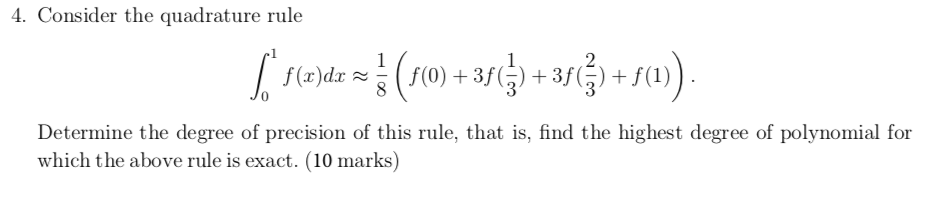4. Consider the quadrature rule +s0) 2 (F'0) +35() + 3fj f (x)dx Determine the degree of precision of this rule, that is, find the highest degree of polynomial for which the above rule is exact. (10 marks) OC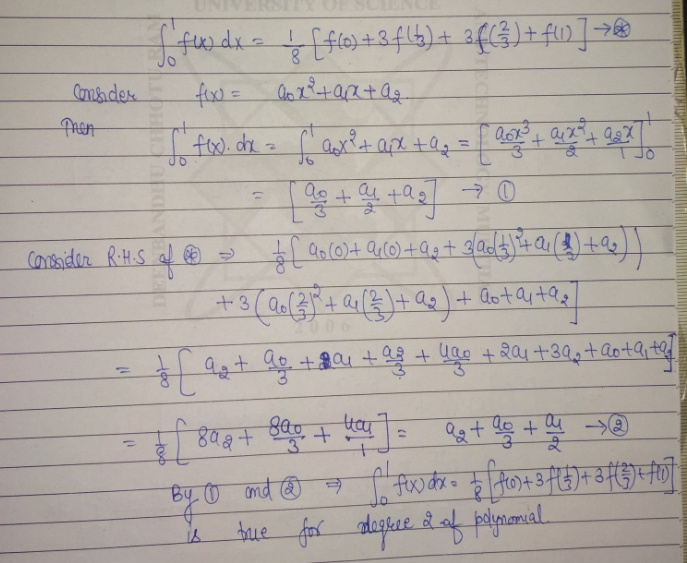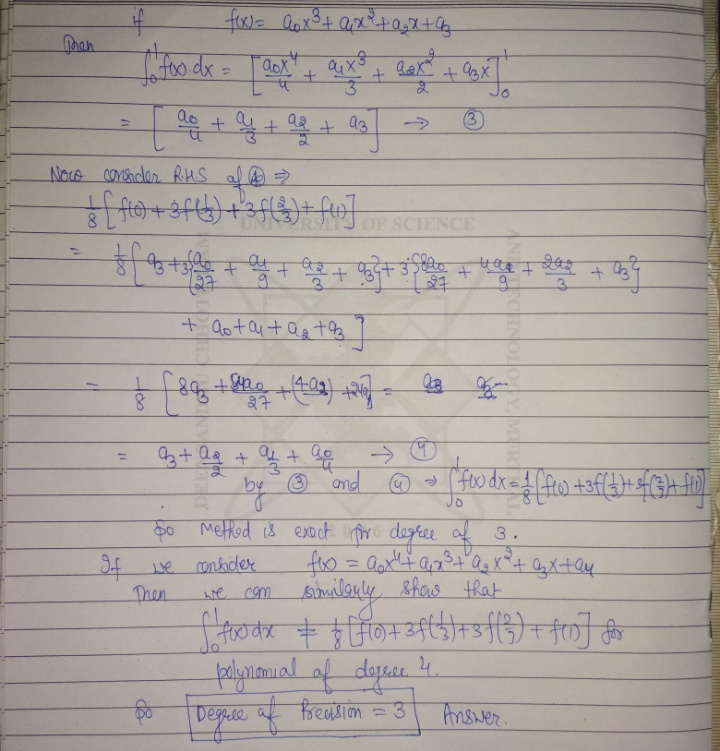##### Add Answer of: 4. Consider the quadrature rule +s0) 2 (F'0) +35() + 3fj f (x)dx Determine the degree...
Similar Homework Help Questions
• ### Problem 1. Consider an integral of the form 1 (f) = f0 /if (x) dx. Show that the one-point Gaussian quadrature rule for integrals of the form Jo VRf (x) dx has the node x1and weight w3 Probl...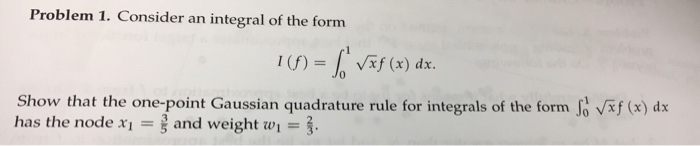Problem 1. Consider an integral of the form 1 (f) = f0 /if (x) dx. Show that the one-point Gaussian quadrature rule for integrals of the form Jo VRf (x) dx has the node x1and weight w3 Problem 1. Consider an integral of the form 1 (f) = f0 /if (x) dx. Show that the one-point Gaussian quadrature rule for integrals of the form Jo VRf (x) dx has the node x1and weight w3

• ### (4.2) Consider the integral -f 1 J dec 1+3 (a) Show that (4) 1 da 1 +x3 dr 1+r3 (b) Deduce that (3) -re) J f() dr wh...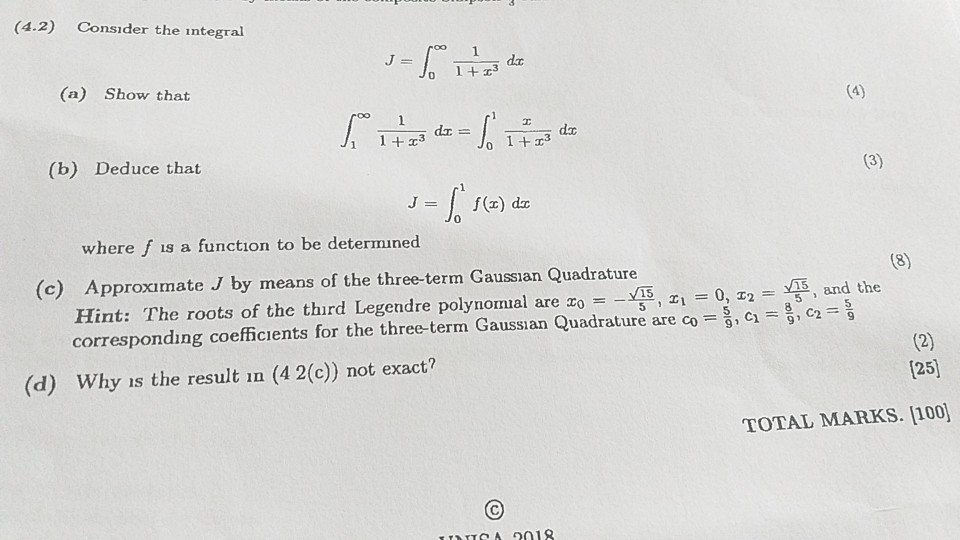(4.2) Consider the integral -f 1 J dec 1+3 (a) Show that (4) 1 da 1 +x3 dr 1+r3 (b) Deduce that (3) -re) J f() dr where f is a function to be determined (8) and the (c) Approximate J by means of the three-term Gaussian Quadrature Hint: The roots of the third Legendre polynomial are xo corresponding coefficients for the three-term Gaussian Quadrature are co =,C= , C2= 15 15, 1 0, 32 5 Y 9 (2) (25]...

• ### Consider the function f(x) := v/x= x1/2. 6. (a) Give the Taylor polynomial P(x) of degree 5 about a1 of this function (b) Give the nested representation of the polynomial Qs()Ps((t)) where t -1 (...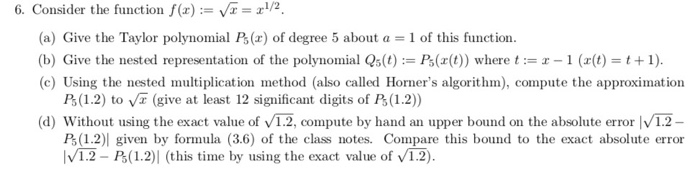Consider the function f(x) := v/x= x1/2. 6. (a) Give the Taylor polynomial P(x) of degree 5 about a1 of this function (b) Give the nested representation of the polynomial Qs()Ps((t)) where t -1 ((t)+1). (c) Using the nested multiplication method (also called Horner's algorithm), compute the approximation Ps (1.2) to V (give at least 12 significant digits of P(1.2)) (d) Without using the exact value of 12, compute by hand an upper bound on the absolute error V1.2 A(1.21...

• ### Question 1 (Quadrature) [50 pts I. Recall the formula for a (composite) trapezoidal rule T, (u) for 1 = u(a)dr whic...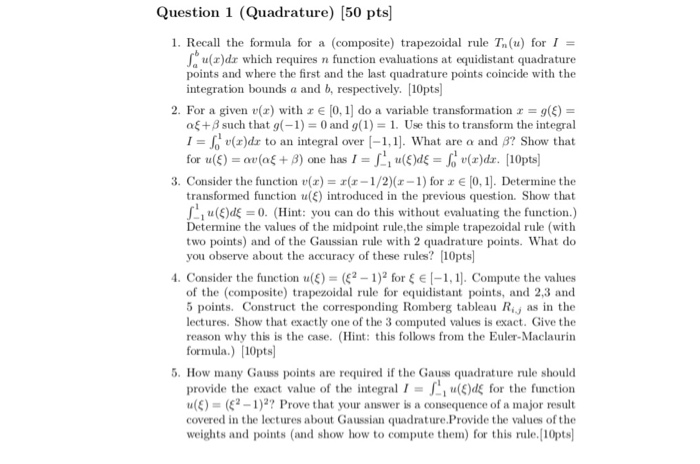Question 1 (Quadrature) [50 pts I. Recall the formula for a (composite) trapezoidal rule T, (u) for 1 = u(a)dr which requires n function evaluations at equidistant quadrature points and where the first and the last quadrature points coincide with the integration bounds a and b, respectively. 10pts 2. For a given v(r) with r E [0,1] do a variable transformation g() af + β such that g(-1)-0 and g(1)-1. Use this to transform the integral に1, u(z)dz to an...

• ### Problem 2 (35 points): Consider function f(x)-1/1) around zo 0 on the interval (0,0.5). (a) Find ...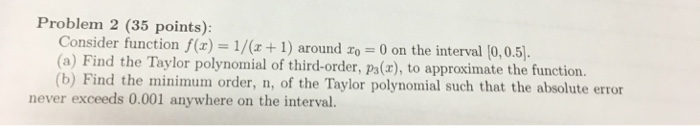Problem 2 (35 points): Consider function f(x)-1/1) around zo 0 on the interval (0,0.5). (a) Find the Taylor polynomial of third-order, pa(x), to approximate the function. (b) Find the minimum order, n, of the Taylor polynomial such that the absolute error never exceeds 0.001 anywhere on the interval. Problem 2 (35 points): Consider function f(x)-1/1) around zo 0 on the interval (0,0.5). (a) Find the Taylor polynomial of third-order, pa(x), to approximate the function. (b) Find the minimum order, n,...

• ### (a) Find the degree 1 interpolating P(x) through the points (a, f(a)) and (b, f(b)) (b) Develop the following formula b...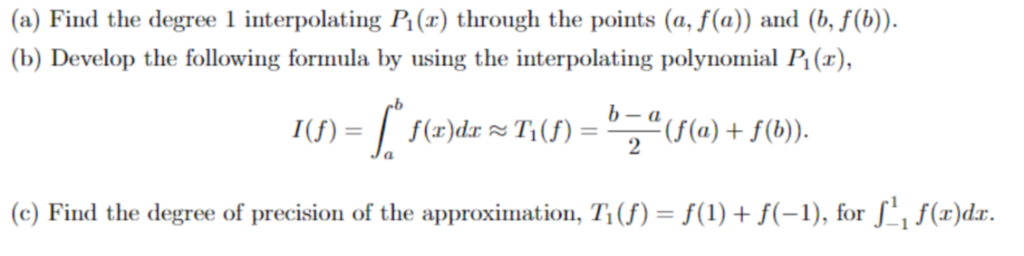(a) Find the degree 1 interpolating P(x) through the points (a, f(a)) and (b, f(b)) (b) Develop the following formula by using the interpolating polynomial P1 (x), (c) Find the degree of precision of the approximation, T1 (f) = f(1) + f(-1), for f(r)dr. (a) Find the degree 1 interpolating P(x) through the points (a, f(a)) and (b, f(b)) (b) Develop the following formula by using the interpolating polynomial P1 (x), (c) Find the degree of precision of the approximation,...

• ### Problem 3. Suppose you are programming the composite trapezoid rule (CTR) to approximate 1(f) =| f(x) dx using the TR with N subintervals, and that you mistakenly forget to weight down the two en...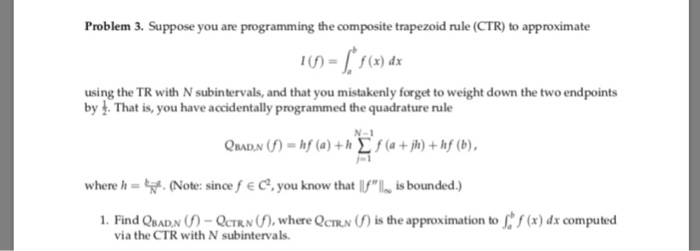Problem 3. Suppose you are programming the composite trapezoid rule (CTR) to approximate 1(f) =| f(x) dx using the TR with N subintervals, and that you mistakenly forget to weight down the two endpoints by 3. That is, you have accidentally programmed the quadrature rule where h-%.. (Note: sinoefe C, you know that UIL is bounded.) 1. Find QBADN -OCTRN where QCTRN ) is the approximation to (x) dx computed via the CTR with N subintervals. Problem 3. Suppose you...

• ### 3. Consider the function()x(x-1/2)(1) for z E 0,1]. Determine the transformed function u() introduced in the previous q...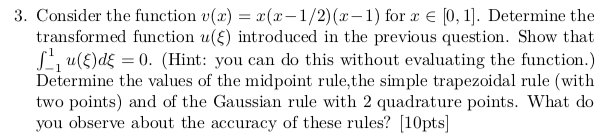3. Consider the function()x(x-1/2)(1) for z E 0,1]. Determine the transformed function u() introduced in the previous question. Show that | -1 u(E)dE-: 0. (Hint: you can do this without evaluating the function.) Determine the values of the midpoint rule,the simple trapezoidal rule (with two points) and of the Gaussian rule with 2 quadrature points. What do you observe about the accuracy of these rules? [10pts] 3. Consider the function()x(x-1/2)(1) for z E 0,1]. Determine the transformed function u() introduced...

• ### T F(x)=∫x0sin(7t2) dt. Find the MacLaurin polynomial of degree 7 for F(x). 7/3x^3-49/6x^7 Use thi...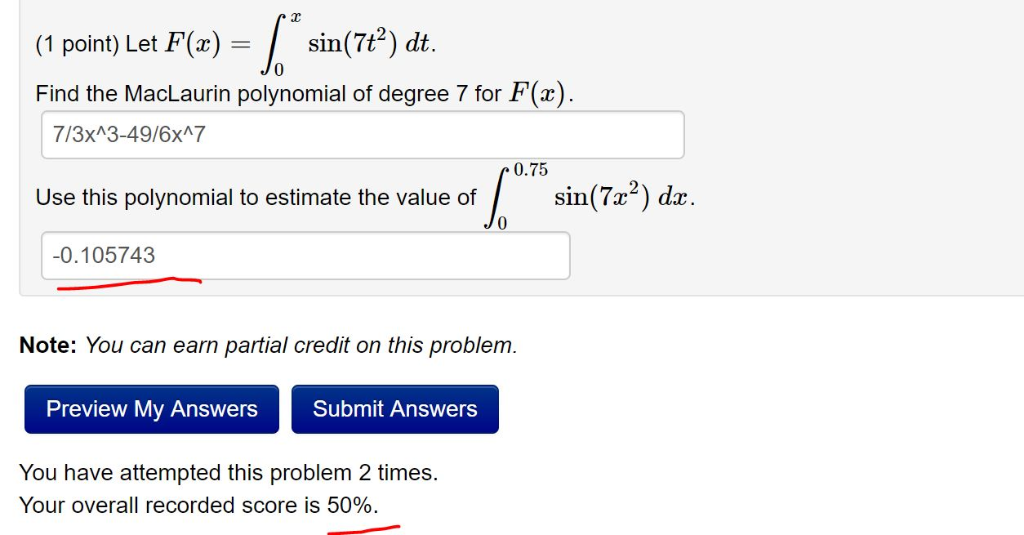t F(x)=∫x0sin(7t2) dt. Find the MacLaurin polynomial of degree 7 for F(x). 7/3x^3-49/6x^7 Use this polynomial to estimate the value of ∫0.750sin(7x2) dx. -0.105743 (1 point) Let F(x)sin(7t2) dt. Find the MacLaurin polynomial of degree 7 for F(x) 713xA3-49/6x7 0.75 Use this polynomial to estimate the value of sin(7x2) dx 0.105743 Note: You can earn partial credit on this problem Preview My Answers Submit Answers You have attempted this problem 2 times. Your overall recorded score is 50%. (1 point)...

• ### Problem 4 Let V be the vector space of functions of the form f(x) = e-xp(x), where p(x) is a polynomial of degree (a) Find the matrix of the derivative operator D = d/dx : V → V in the basis ek = e-x...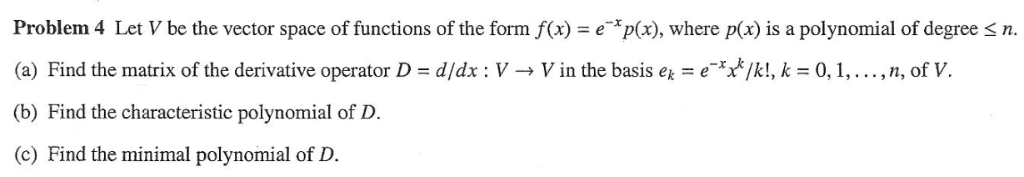Problem 4 Let V be the vector space of functions of the form f(x) = e-xp(x), where p(x) is a polynomial of degree (a) Find the matrix of the derivative operator D = d/dx : V → V in the basis ek = e-xXk/k!, k = 0, 1, . .. , n, of V. (b) Find the characteristic polynomial of D. (c) Find the minimal polynomial of D n. Problem 4 Let V be the vector space of functions of...

Need Online Homework Help?